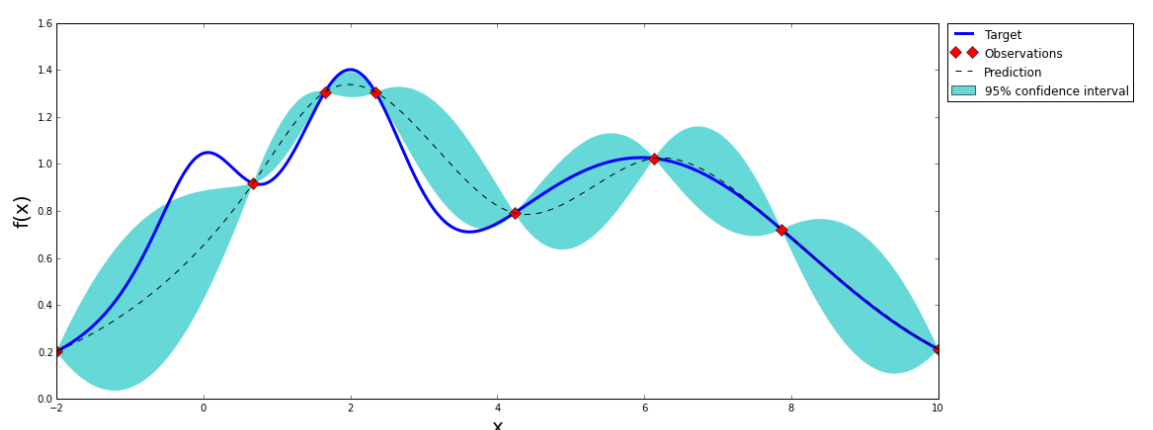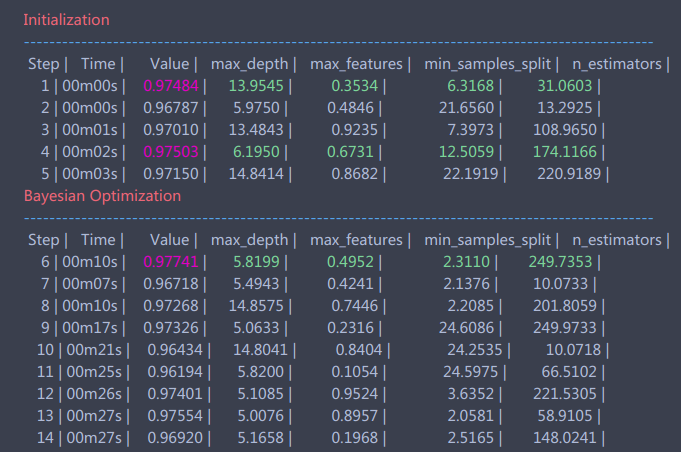# 强大而精致的机器学习调参方法：贝叶斯优化

## 一、简介

• 贝叶斯调参采用高斯过程，考虑之前的参数信息，不断地更新先验；网格搜索未考虑之前的参数信息
• 贝叶斯调参迭代次数少，速度快；网格搜索速度慢,参数多时易导致维度爆炸
• 贝叶斯调参针对非凸问题依然稳健；网格搜索针对非凸问题易得到局部优最

## 二、理论

• 高斯过程，用以拟合优化目标函数
• 贝叶斯优化，包括了“开采”和“勘探”，用以花最少的代价找到最优值

## 2.1 高斯过程

$p(y_{N+1} | X_{N+1})$

$p(y_{N+1} | X_{N+1}, y_{N})$

$\begin{bmatrix} y_1 \\ y_2 \\ ... \\ y_n \\ \end{bmatrix} \sim N( \mathbf{0}, \begin{bmatrix} k(x_1, x_1) , k(x_1, x_2), ..., k(x_1, x_n) \\ k(x_2, x_1) , k(x_2, x_2), ..., k(x_2, x_n) \\ ... \\ k(x_n, x_1) , k(x_n, x_2), ..., k(x_n, x_n) \end{bmatrix} )$

$p(y_*| \mathbf{y} \sim N(K_* K^{-1} \mathbf{y}, ~ K_{**} - K_* K^{-1} K_*^T)$

$\begin{bmatrix} \mathbf{y} \\ y_* \end{bmatrix} \sim N(\mathbf{0}, \begin{bmatrix} K, K_*^T \\ K_*, K_{**} \\ \end{bmatrix})$

### 高斯过程的估计（训练）方法

$k(x_1, x_2) = \sigma^2_f exp(\frac{-(x_1 - x_2)^2}{2 l^2})$

$L = log p(y| x, \theta) = - \frac{1}{2} log|\mathbf{K}| - \frac{1}{2} (y - \mu)^T \mathbf{K}^{-1} (y - \mu) - n*log(2\pi)/2$## 2.2 贝叶斯优化理论

### 基本思想

• 开发 (exploitation)： 根据后验分布，在最可能出现全局最优解的区域进行采样, 开发高意味着均值高
• 探索 (exploration): 在还未取样的区域获取采样点， 探索高意味着方差高

### Acquistion Function

#### POI(probability of improvement)

$POI(X) = P(f(X) \ge f(X^+) + \xi) = \Phi(\frac{\mu(x) - f(X^+) - \xi}{\sigma(x)})$#### Expected Improvement

POI是一个概率函数，因此只考虑了f(x) 比 $f(x^+)$ 大的概率，而EI则是一个期望函数，因此考虑了 f(x) 比 $f(x^+)$ 大多少。我们通过下式获取x

$x = argmax_x \ \ E(\max\{0, f_{t+1}(x) - f(X^+)\}| D_t)$

$EI(x) = \begin{cases} (\mu(x) - f(x^+)) \Phi(Z) + \sigma(x) \phi(Z), if \ \sigma(x) > 0 \\ 0, if \ \sigma(x) = 0 \end{cases}$

#### Confidence bound criteria

$LCB(x) = \mu(x) - \kappa \sigma(x)$

$UCB(x) = \mu(x) + \kappa \sigma(x)$

• 高斯过程核矩阵不好选

# 三、例子

## 安装

pip install bayesian-optimization

## 前期准备

from sklearn.datasets import make_classification
from sklearn.ensemble import RandomForestClassifier
from sklearn.cross_validation import cross_val_score
from bayes_opt import BayesianOptimization

# 产生随机分类数据集，10个特征， 2个类别
x, y = make_classification(n_samples=1000,n_features=10,n_classes=2)

rf = RandomForestClassifier()
print(np.mean(cross_val_score(rf, x, y, cv=20, scoring='roc_auc')))

>>> 0.965162

## bayes调参初探

def rf_cv(n_estimators, min_samples_split, max_features, max_depth):
val = cross_val_score(
RandomForestClassifier(n_estimators=int(n_estimators),
min_samples_split=int(min_samples_split),
max_features=min(max_features, 0.999), # float
max_depth=int(max_depth),
random_state=2
),
x, y, scoring='roc_auc', cv=5
).mean()
return val


 rf_bo = BayesianOptimization(
rf_cv,
{'n_estimators': (10, 250),
'min_samples_split': (2, 25),
'max_features': (0.1, 0.999),
'max_depth': (5, 15)}
)

rf_bo.maximize()rf_bo.res['max']

>>> {'max_params': {'max_depth': 5.819908283575526,
'max_features': 0.4951745603509127,
'min_samples_split': 2.3110014720414958,
'n_estimators': 249.73529231990733},
'max_val': 0.9774079407940794}

## bayes调参进阶


rf_bo.explore(
{'n_estimators': [10, 100, 200],
'min_samples_split': [2, 10, 20],
'max_features': [0.1, 0.5, 0.9],
'max_depth': [5, 10, 15]
}
)

gp_param={'kernel':None}
rf_bo.maximize(**gp_param)

{'max_params': {'max_depth': 5.819908283575526,
'max_features': 0.4951745603509127,
'min_samples_split': 2.3110014720414958,
'n_estimators': 249.73529231990733},
'max_val': 0.9774079407940794}

rf = RandomForestClassifier(max_depth=6, max_features=0.39517, min_samples_split=2, n_estimators=250)
np.mean(cross_val_score(rf, x, y, cv=20, scoring='roc_auc'))
>>> 0.9754953

# Reference

posted @ 2018-07-30 21:49 杨睿 阅读(...) 评论(...) 编辑 收藏